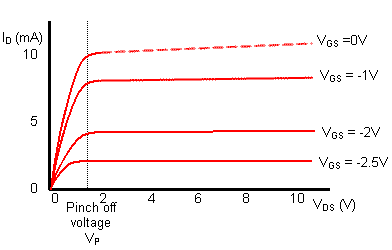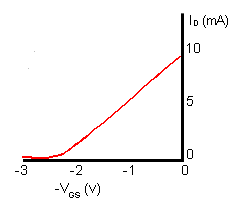# How a JFET Works

• Section 4.2 How a JFET Works.
• • Operation Below Pinch Off.
• • Operation Above Pinch Off.
• • JFET Output Characteristic.
• • JFET Transfer Characteristic.
• • JFET Video.

## The JFET is a Voltage Controlled Transistor.

The JFET is a voltage controlled transistor that has two distinct areas of operation depending on the whether the voltage applied to the Source and Drain terminals is greater or less that the transistor's Pinch-Off Voltage

## The Pinch Off Voltage

The Pinch-Off value of the JFET refers to the voltage applied between Drain and Source (with the Gate voltage at zero volts) at which maximum current flows. Operating with the Drain/Source voltage below this value is classed is the "Ohmic Region" as the JFET will act rather like a resistor. Operating with the Drain Source voltage above Pinch Off is known as the "Saturation Region" as the JFET is acting like a saturated transistor; that is any increase in voltage does not produce a relative increase in current.

## Operation Below Pinch Off### Fig. 4.2.1 JFET Operation Below "Pinch Off"

In the planar construction N channel JFET shown in Fig. 4.2.1, the N channel is sandwiched between two P type regions (the gate and the substrate) that are connected together and are at 0V. This forms the gate. The N type channel is connected to the source and drain terminals via more heavily doped N+ type regions. The drain is connected to a positive supply, and the source to zero volts. N+ type silicon has a lower resistivity than N type. This gives it a lower resistance, increasing conduction and reducing the effect of placing standard N type silicon next to the aluminium connector, which because aluminium is a tri-valent material, having three valence electrons whilst silicon has four, would tend to create an unwanted junction, similar in effect to a PN junction at this point.

The P type gate is at 0V and is therefore negatively biased compared to the channel, which has a potential gradient on it, as one end is connected to 0 volts (the source), and the other end to a positive voltage (the drain). Any point on the channel (apart from the extreme end near the source terminal) must therefore be more positive than the gate. Therefore the two PN junctions formed between the N type conducting channel and the P type areas of the gate/substrate are both reverse biased, and so have a depletion layer that extends into the channel as shown in Fig. 4.2.1.

The shape of the depletion layer is not symmetrical, as can be seen from Fig. 4.2.1. It is generally thicker towards the drain end of the channel, because the voltage on the drain is more positive than that on the source due to the voltage gradient that exists along the channel. This causes a larger potential across the junctions nearer the drain, and so a thickening of the depletion layer. The effect becomes more marked when the voltage between drain and source is greater than about 1 volt or so.

## Operation Above "Pinch Off"### Fig. 4.2.2 JFET Operation Above "Pinch Off"

When a voltage is applied between drain and source (VDS) current flows and the silicon channel acts rather like a conventional resistor (The Ohmic Region). Now if VDS is increased (with VGS held at zero volts) towards what is called the pinch off value VP, the drain current ID also at first, increases. The transistor is working in the "ohmic region" as shown in Fig. 4.2.1.

However as drain source voltage VDS increases, the depletion layers at the gate junctions are also becoming thicker and so narrowing the N type channel available for conduction. There comes a point, (Pinch Off) where the conducting channel has become narrow enough to cancel out the effect of current increasing with the applied voltage VDS as shown in fig 4.2.2. Above this (Pinch Off) point there is little further increase in drain current and the transistor is said to operating in the "Saturation Region".

However, if the JFET is biased with VDS at Pinch Off voltage (VP) a small change in VGS can be used to control the current through the source-drain channel from zero current to its maximum (saturated)value .

## JFET Characteristics### Fig. 4.2.3 JFET Output Characteristic

This type of operation is shown in the fairly flat top to the output characteristics shown in Fig 4.2.3. Notice that each curve is drawn for a particular value of negative voltage between gate and source, and that when sufficient reverse bias is applied to the gate (e.g. more than -2.5V, the lowest value on the graph) the drain current ceases completely.

In the JFET output characteristics shown in Fig. 4.2.3, the Drain current ID increases in a linear manner (like a resistor) at values of Gate/Source voltage (VGS) below pinch off (The Ohmic Region), but above VP (The Saturation Region) shows very little change, and the curves are very nearly horizontal at voltages greater than the pinch off voltage (VP). Almost all of the expected increase in current, due to the increase in voltage between Source and Drain (VDS), is offset by the narrowing of the conducting channel due to the growing depletion layers.### Fig. 4.2.4 JFET Transconductance Characteristic

The tranconductance characteristic for a JFET, which shows the change in Drain current (ID) for a given change in Gate-Source voltage (VGS), is shown in Fig 4.2.4. Because the JFET input (the Gate) is voltage operated, the gain of the transistor cannot be called current gain, as with bipolar transistors. The drain current is controlled by the Gate-Source voltage, so the graph shows milli-amperes per volt (mA / V), and as current divided by Voltage (I/V) is CONDUCTANCE (the inverse of resistance (V/I) the slope of this graph (the gain of the device) is called the FORWARD or MUTUAL TRANSCONDUCTANCE, which has the symbol gm. Therefore the higher the value of gm the greater the amplification.

Notice that VGS is always shown as being negative; in reality it may be zero or slightly above zero, but the gate is always more negative than the N type channel between source and drain. Note also that the slope of the curve in the transfer characteristic is less steep than that of the Mutual Conductance characteristic for a typical bipolar transistor (compare Fig. 4.2.4 and Fig. 3.5.4 on the Bipolar Transistors Current Gain page). This means that a JFET will have a lower gain than that of a bipolar transistor.

This disadvantage is offset by the advantage of having an extremely high input resistance. A typical input resistance for a JFET would be in the region of 1 x 1010 ohms (10,000 Megohms!) compared with 2K to 3K Ohms for a bipolar device.

This makes the JFET ideal for applications where the circuit or device driving the JFET amplifier cannot supply any appreciable current, an example being the Electret microphone, which uses a FET within the microphone to amplify the tiny voltage variations appearing across the vibrating diaphragm element.

Another feature of the JFET that makes it more suited to very high frequency use than bipolar transistors, is the absence of junctions in the JFET conducting channel. In a bipolar transistor two PN junctions forming tiny capacitances, exist between base and emitter, and base and collector, due to the PN junctions. These capacitances will limit high frequency performance, as they provide negative feedback paths at high frequencies. Because the JFET is in effect just a slab of silicon between Source and Drain, the stray capacitances that exist in bipolar devices are absent, so high frequency performance is improved, making JFETs usable even at hundreds of MHz.

Download a datasheet for a typical N Channel JFET from On Semiconductor

Top of Page.>